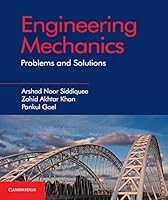# Engineering Mechanics: Problems and Solutions## Book Description

This comprehensive and self-contained textbook will help students in acquiring an understanding of fundamental concepts and applications of mechanics. With basic prior knowledge, the readers are guided through important concepts of mechanics such as free body diagrams, of the transmissibility of forces, Coulomb's of friction, of forces in members of truss and rectilinear motion in horizontal direction. Important theorems including Lami's theorem, Varignon's theorem, axis theorem and perpendicular axis theorem are discussed in a step-by-step manner for better clarity. Applications of ladder friction, wedge friction, screw friction and belt friction are discussed in detail. The textbook is primarily written for undergraduate engineering students in India. Numerous theoretical questions, unsolved numerical problems and solved problems are included throughout the text to develop a clear understanding of the key principles of engineering mechanics. This text is the ideal resource for first year engineering undergraduates taking an introductory, single-semester course in engineering mechanics.